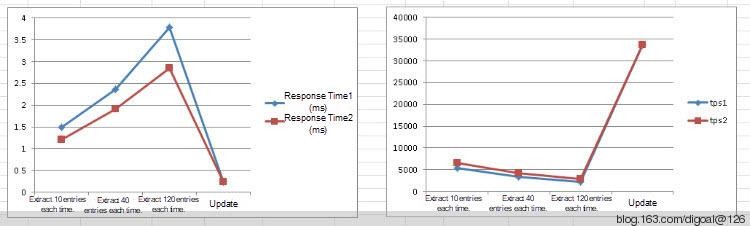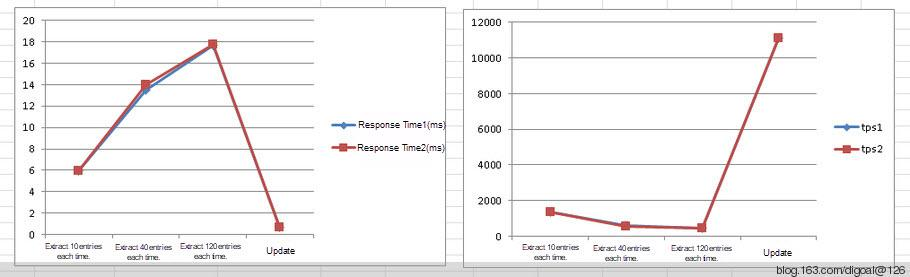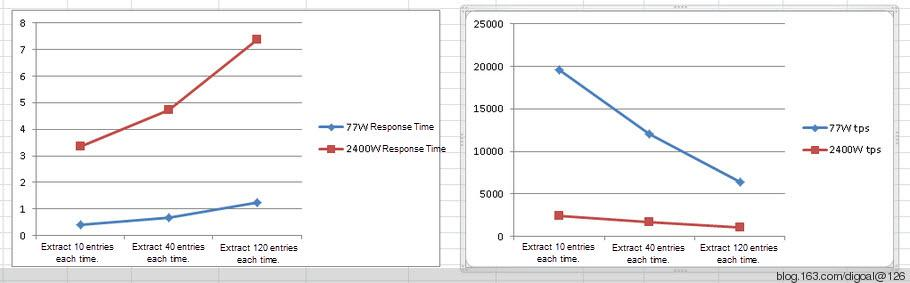# Community

Blog Events Webinars Tutorials Forum
×
Community Blog Sorting GiST Indexes by Leveraging Nearest-neighbor Enhancement in PostgreSQL 9.1

# Sorting GiST Indexes by Leveraging Nearest-neighbor Enhancement in PostgreSQL 9.1

This article discusses the nearest-neighbor enhancement added in PostgreSQL 9.1 for sorting GiST indexes with sample code and examines the performance using test data.

By Digoal

## Background

PostgreSQL 9.1 has added the nearest-neighbor enhancement to sort GiST indexes using the ORDER BY operator.

Example:

``````digoal=>  create table gist_test (id serial primary key,location point);
NOTICE:  CREATE TABLE will create implicit sequence "gist_test_id_seq" for serial column "gist_test.id"
NOTICE:  CREATE TABLE / PRIMARY KEY will create implicit index "gist_test_pkey" for table "gist_test"
CREATE TABLE
Time: 40.290 ms
digoal=> insert into gist_test (location) select cast ('(1,'||generate_series(1,1000000)||')' as point);
INSERT 0 1000000
Time: 11143.666 ms
digoal=> create index idx_gist_test_1 on gist_test using gist (location point_ops);
CREATE INDEX
Time: 14874.948 ms``````

In the following operation, search results will be scanned and returned by index, which is highly efficient.

``````digoal=> select * from gist_test order by location <-> cast ('(1,2)' as point) limit 10;
id | location
----+----------
2 | (1,2)
3 | (1,3)
1 | (1,1)
4 | (1,4)
5 | (1,5)
6 | (1,6)
7 | (1,7)
8 | (1,8)
9 | (1,9)
10 | (1,10)
(10 rows)
Time: 0.716 ms
digoal=> explain verbose select * from gist_test order by location <-> cast ('(1,2)' as point) limit 10;
QUERY PLAN
---------------------------------------------------------------------------------------------------------
Limit  (cost=0.00..0.50 rows=10 width=20)
Output: id, location, ((location <-> '(1,2)'::point))
->  Index Scan using idx_gist_test_1 on digoal.gist_test  (cost=0.00..50374.43 rows=1000000 width=20)
Output: id, location, (location <-> '(1,2)'::point)
Order By: (gist_test.location <-> '(1,2)'::point)
(5 rows)
Time: 0.465 ms``````

Without indexes, the efficiency is as follows:

``````digoal=> drop index idx_gist_test_1 ;
DROP INDEX
Time: 60.281 ms
digoal=> explain verbose select * from gist_test order by location <-> cast ('(1,2)' as point) limit 10;
QUERY PLAN
---------------------------------------------------------------------------------------
Limit  (cost=40479.64..40479.67 rows=10 width=20)
Output: id, location, ((location <-> '(1,2)'::point))
->  Sort  (cost=40479.64..42979.64 rows=1000000 width=20)
Output: id, location, ((location <-> '(1,2)'::point))
Sort Key: ((gist_test.location <-> '(1,2)'::point))
->  Seq Scan on digoal.gist_test  (cost=0.00..18870.00 rows=1000000 width=20)
Output: id, location, (location <-> '(1,2)'::point)
(7 rows)
Time: 0.613 ms
digoal=> select * from gist_test order by location <-> cast ('(1,2)' as point) limit 10;
id | location
----+----------
2 | (1,2)
3 | (1,3)
1 | (1,1)
4 | (1,4)
5 | (1,5)
6 | (1,6)
7 | (1,7)
8 | (1,8)
9 | (1,9)
10 | (1,10)
(10 rows)
Time: 437.322 ms``````

With indexes, the efficiency improves significantly.

The <-> operator is applicable in the following scenarios:

``````digoal=> create or replace function get_type(i_oid oid) returns text as \$\$
declare
v_type text;
begin
select typname into v_type from pg_type where oid=i_oid limit 1;
return v_type;
end;
\$\$ language plpgsql;

digoal=> select oprname,get_type(oprleft),get_type(oprright) from pg_operator where oprname='<->';
oprname | get_type | get_type
---------+----------+----------
<->     | point    | point
<->     | point    | lseg
<->     | point    | path
<->     | point    | box
<->     | point    | line
<->     | point    | circle
<->     | lseg     | lseg
<->     | lseg     | box
<->     | lseg     | line
<->     | path     | path
<->     | box      | box
<->     | polygon  | polygon
<->     | line     | box
<->     | line     | line
<->     | circle   | polygon
<->     | circle   | circle
(16 rows)``````

Currently, the KNN algorithm is used.

If the raw output has an acceptable error rate, reordering is not recommended because reordering will increase overhead. I have tested this operator on Momo and detected that the output also has a moderate error rate, which is not corrected.

For example, the following result of ORDER BY geometry <-> geometry produces an error:

``````  user_id  | st_distance_sphere
-----------+--------------------
105137620 |                  0
302151638 |   17.1253091983443
284707523 |   297.073719657173
305881734 |   468.361916333953
304983707 |   493.019043438912
283249783 |   861.119017114206
295055791 |   861.135253407573
303738892 |   734.735620683819
283827579 |   734.735620683819
283394455 |   772.808877940454
300115846 |   943.892953926387
277775985 |   1082.41544076904  ``````

## Introduction to the <-> Operator

<-> : returns the distance between two points.

It uses floating-point accuracy for point/point-checks (as opposed to the double-precision accuracy of the underlying point geometry).

For other geometry types, the distance between the floating-point bounding box centroids is returned.

It is useful for doing distance ordering and nearest neighbor limits using KNN gist functionality.

The SQL statement for creating the operator is as follows:

``````postgis/postgis.sql.in.c

#if POSTGIS_PGSQL_VERSION >= 91
CREATE OPERATOR <-> (
LEFTARG = geometry, RIGHTARG = geometry, PROCEDURE = geometry_distance_centroid,
COMMUTATOR = '<->'
); ``````

The following function is used:

``````-- Availability: 2.0.0
CREATE OR REPLACE FUNCTION geometry_distance_centroid(geom1 geometry, geom2 geometry)
RETURNS float8
AS 'MODULE_PATHNAME' ,'gserialized_distance_centroid_2d'
LANGUAGE 'c' IMMUTABLE STRICT;
Source code:
postgis/gserialized_gist_2d.c
/***********************************************************************
* GiST 2-D Index Operator Functions
*/

PG_FUNCTION_INFO_V1(gserialized_distance_centroid_2d);
Datum gserialized_distance_centroid_2d(PG_FUNCTION_ARGS)
{
BOX2DF b1, b2;
Datum gs1 = PG_GETARG_DATUM(0);
Datum gs2 = PG_GETARG_DATUM(1);

POSTGIS_DEBUG(3, "entered function");

/* Must be able to build box for each argument (ie, not empty geometry). */
if ( (gserialized_datum_get_box2df_p(gs1, &b1) == LW_SUCCESS) &&
(gserialized_datum_get_box2df_p(gs2, &b2) == LW_SUCCESS) )
{
double distance = box2df_distance_leaf_centroid(&b1, &b2);
POSTGIS_DEBUGF(3, "got boxes %s and %s", box2df_to_string(&b1), box2df_to_string(&b2));
PG_RETURN_FLOAT8(distance);
}
PG_RETURN_FLOAT8(MAXFLOAT);
}  ``````

The following lists the test data of querying nearby user information by using PostGIS:

Test environment:

``````CentOS 5.x 64bit
PostgreSQL 9.1
PostGIS 2.0.1
MEM 8GB*12
CPU 2 * Intel(R) Xeon(R) CPU E5504  @ 2.00GHz  ``````

Test results in scenario 1 with over 770,000 data entries:Test results in scenario 2 with over 24,750,000 data entries:## Summary

1) Bottleneck: CPU

2) The performance varies according to the data volume. The data retrieve speed in a region where data is distributed sparsely is obviously slower than that in a data-intensive region.

3) There is an error rate of about 10% between the sorting result and the actual distance.

4) Optimization for future reads: 1. SQL 2. CPU 3. Data replication

5) Optimization for future writes: 1. SQL 2. CPU 3. Database sharding

6) Update optimization: If the difference between the location uploaded by a user and the location stored in the database is within 10 meters, then the record in the database does not need to be updated. This can reduce the number of update requests.

7) Request optimization: The distance computation operation is performed in the app but not in the database. This reduces the operation overhead of the database and increases the response speed by 12.7%.

8) Add a Point column and use the cast(replace(substr(ST_AsText(lonlat),6),' ',',') as point) function to convert geometries into points. For non-boundary users, nearby user information is extracted by point. This is because data extraction by point is obviously faster than data extraction by geometry. For boundary users, nearby user data is extracted by geometry. After obtaining location information, the application needs to distinguish boundary users from non-boundary users and use different methods accordingly.

## Detailed Test Data

### 1) Data Volume: 773,632 Entries

Read 10 nearby user information entries each time:

1) Extract skyids and distances.

The average response time is 1.50 milliseconds, and 5,318 requests are processed per second. The CPU usage is 100%.

2) Extract skyids and geographic locations, with distances calculated by the application.

The average response time is 1.21 milliseconds, and 6,568 requests are processed per second. The CPU usage is 100%.

Read 40 nearby user information entries each time:

1) Extract skyids and distances.

The average response time is 2.36 milliseconds, and 3,380 requests are processed per second. The CPU usage is 100%.

2) Extract skyids and geographic locations, with distances calculated by the application.

The average response time is 1.91 milliseconds, and 4,167 requests are processed per second. The CPU usage is 100%.

Read 120 nearby user information entries each time:

1) Extract skyids and distances.

The average response time is 3.78 milliseconds, and 2,109 requests are processed per second. The CPU usage is 100%.

2) Extract skyids and geographic locations, with distances calculated by the application.

The average response time is 2.85 milliseconds, and 2,795 requests are processed per second. The CPU usage is 100%.

Geographic location update test:

The average response time is 0.23 millisecond, and 33,621 requests are processed per second. The CPU usage is 90%.

### 2) Data Volume: 24,756,224 Entries

Read 10 nearby user information entries each time:

1) Extract skyids and distances.

The average response time is 5.98 milliseconds, and 1,336 requests are processed per second. The CPU usage is 100%.

2) Extract skyids and geographic locations, with distances calculated by the application.

The average response time is 5.97 milliseconds, and 1,338 requests are processed per second. The CPU usage is 100%.

Read 40 nearby user information entries each time:

1) Extract skyids and distances.

The average response time is 13.5 milliseconds, and 593 requests are processed per second. The CPU usage is 100%.

2) Extract skyids and geographic locations, with distances calculated by the application.

The average response time is 14.0 milliseconds, and 571 requests are processed per second. The CPU usage is 100%.

Read 120 nearby user information entries each time

1) Extract skyids and distances.

The average response time is 17.7 milliseconds, and 451 requests are processed per second. The CPU usage is 100%.

2) Extract skyids and geographic locations, with distances calculated by the application.

The average response time is 17.8 milliseconds, and 449 requests are processed per second. The CPU usage is 100%.

Geographic location update test:

The average response time is 0.71 millisecond, and 11,100 requests are processed per second. The CPU usage is 90%.

Another optimization method is to convert geometries into points and sort the points. Details are as follows:

The territory of China spans across only positive longitudes and latitudes and is at a distance from boundaries. Therefore, it is feasible to measure distances by points.

The northernmost point is located at the center line of the Heilongjiang River's main channel, north of the town of Mohe in Heilongjiang Province, at a latitude of 53° N.

The southernmost point is located at Zengmu Reef in the southern South China Sea, at a latitude of 4° N.

The easternmost point is located at the intersection of the center lines of the Heilongjiang River's main channels and the Wusuli River, at a longitude of 135° E.

The westernmost point is located on the Pamir Plateau, at a longitude of 73° E.

If geometries are converted into points, the results of distance sorting by point is the same as by geometry, and the performance of extracting nearby user information is greatly improved.

``````urslbs_test=> alter table test1 add column col1 point;
urslbs_test=> update test1 set col1 = cast(replace(substr(ST_AsText(lonlat),6),' ',',') as point);
urslbs_test=> create index idx_test1_col1 on test1 using gist(col1);
urslbs_test=> select cast(replace(substr(ST_AsText('0101000020E61000002FC786C9AF2A5D40866BFF14D8254440'::geometry(Point,4326)),6),' ',',') as point);
replace
--------------------------
(116.6669792,40.2956568)
(1 row)
urslbs_test=> select point_distance(col1,'(116.6669792,40.2956568)'::point), st_distance_sphere('0101000020E61000002FC786C9AF2A5D40866BFF14D8254440'::geometry(Point,4326), lonlat) from test1 order by col1 <-> '(116.6669792,40.2956568)'::point limit 10;
point_distance    | st_distance_sphere
----------------------+--------------------
0 |                  0
0.000154592140808128 |   17.1253091983443
0.00336883988488212 |   297.073719657173
0.00490960806990255 |   468.361916333953
0.0049619458693147 |   493.019043438912
0.00786551161781989 |   861.135253407573
0.00786595648666547 |   861.119017114206
0.00792924406107087 |   734.735620683819
0.00792924406107087 |   734.735620683819
0.00817754802614712 |   772.808877940454
(10 rows)

urslbs_test=> select st_distance_sphere('0101000020E61000002FC786C9AF2A5D40866BFF14D8254440'::geometry(Point,4326), lonlat) from test1 order by lonlat <-> '0101000020E61000002FC786C9AF2A5D40866BFF14D8254440'::geometry(Point,4326) limit 10;
st_distance_sphere
--------------------
0
17.1253091983443
297.073719657173
468.361916333953
493.019043438912
861.135253407573
861.119017114206
734.735620683819
734.735620683819
772.808877940454
(10 rows)  ``````## Detailed Test Data

### 1) Data Volume: 773,632 Entries

Read test (extract skyids and geographic locations, with distances calculated by the application):

Read 10 nearby user information entries each time:

The average response time is 0.40 millisecond, and 19,573 requests are processed per second. The CPU usage is 100%.

Read 40 nearby user information entries each time:

The average response time is 0.66 millisecond, and 12,004 requests are processed per second. The CPU usage is 100%.

Read 120 nearby user information entries each time:

The average response time is 1.24 milliseconds, and 6,444 requests are processed per second. The CPU usage is 100%.

### 2) Data Volume: 24,756,224 Entries

Read test (extract skyids and geographic locations, with distances calculated by the application):

Read 10 nearby user information entries each time:

The average response time is 3.34 milliseconds, and 2,390 requests are processed per second. The CPU usage is 100%.

Read 40 nearby user information entries each time:

The average response time is 4.73 milliseconds, and 1,689 requests are processed per second. The CPU usage is 100%.

Read 120 nearby user information entries each time:

The average response time is 7.38 milliseconds, and 1,082 requests are processed per second. The CPU usage is 100%.

0 0 0
Share on

# Related Products

• ##Database Overview

Fully managed and less trouble database services

• ##AnalyticDB for PostgreSQL

An online MPP warehousing service based on the Greenplum Database open source program

• ##ApsaraDB RDS for PostgreSQL

An on-demand database hosting service for PostgreSQL with automated monitoring, backup and disaster recovery capabilities